## 1) Import required libraries

This section loads the list of requried libraries for plotting and running models

``````library("RColorBrewer")
library("ggplot2")
library("plyr")
library("vegan")
library("reshape2")
library("ape")
library("phyloseq")
library("knitr")
library("xtable")``````

## 2) Import OTU + mapping files

This section loads the list of OTU tables in BIOM format and a mapping table in csv or tab delimited format. Both files are merged to generate a phyloseq file called “comp”

``````#otu files
otu_all = "../Desktop/Thesis_files/R_files/otus.biom"
file<-import_biom(otu_all)
map = sample_data(m)
comp <-merge_phyloseq(file,map)
``````##               Soil Species Individuals     Plate    Comb Native nodC_clade
## master.96.A9   Mic     Mic          39 master_96     Mic    yes          n
## master.96.G7   Bif     Bar          78 master_96 Bif_Bar     no          x
## master.96.C8   Mac     Bar          65 master_96 Mac_Bar     no          j
## master.48.F4   Bar     Bar          20 master_48     Bar    yes          p
## master.96.B2   Mic     Mac          44 master_96 Mic_Mac     no          n
## master.96.G11  Bif     Mic          87 master_96 Bif_Mic     no          x
##               nod_size   X18s_PD    trna_PD elison_PD Description
## master.96.A9       0.6 0.0000000 0.00000000 0.0000000          F5
## master.96.G7       2.3 0.2271033 0.04134491 0.1994908          A2
## master.96.C8       0.6 0.2594373 0.08283154 0.2993784          B5
## master.48.F4       1.0 0.0000000 0.00000000 0.0000000          C6
## master.96.B2       0.2 0.2371527 0.09260847 0.2573638         F10
## master.96.G11      1.0 0.1928626 0.05857074 0.1574762          C4``````
``````colnames(tax_table(comp)) <- c(k = "Kingdom", p = "Phylum", c = "Class", o = "Order", f = "Family", g = "Genus", s = "Species")
#Sample counts
``````## 2496608  783451  213370  968854  839247 4339860
##       4       3      11       1       1       2``````
``````#Complete otu mb
comp``````
``````## phyloseq-class experiment-level object
## otu_table()   OTU Table:         [ 2394 taxa and 144 samples ]
## sample_data() Sample Data:       [ 144 samples by 12 sample variables ]
## tax_table()   Taxonomy Table:    [ 2394 taxa by 7 taxonomic ranks ]``````

## 3) Subsetting abundant and rare microbiomes from the complete OTU table. Rare microbiome consists of OTUs NOT falling under the “Rhizobiales” order

Before we subset the complete OTU table we need to process it to remove mislabelled/ badly assigned OTUs. We drop all OTUs that had no hits in the greengenes database. The cleaned OTU table is stored in “mb_all”

``````#removing low quality taxa matches and normalize
mb_all= subset_taxa(comp, Kingdom!="None" & Kingdom!="NOHIT" )
mb_all``````
``````## phyloseq-class experiment-level object
## otu_table()   OTU Table:         [ 1314 taxa and 144 samples ]
## sample_data() Sample Data:       [ 144 samples by 12 sample variables ]
## tax_table()   Taxonomy Table:    [ 1314 taxa by 7 taxonomic ranks ]``````
``#Dropped 1080 OTUs``

## 4) Begin normalisation

Our OTU tables are distributed with a highly abundant fraction and highly variable albeit less abundantly distributed rare fraction. This code block separates the complete OTU table into abundant and rare OTU tables. We normalise all reads to sample number and library size. Also a distance matrix using bray curtis distances were generated. At the end if thus step we had 3 OTU tables. An abundant OTU table (mb_rhiz) comprising of all OTUs belonging to the Rhizobiales order, a rare OTU table with all the less abundant OTUs (mb_rare) and finally the complete OTU table mb_all with all the OTUs that cleared the processing stage.

``````norm_all = transform_sample_counts(mb_all, function(x) x/sum(x))
df_all = as(sample_data(norm_all), "data.frame")
d_all = phyloseq::distance(norm_all, "bray")
#NO. of OTUs identified in complete microbiome
norm_all``````
``````## phyloseq-class experiment-level object
## otu_table()   OTU Table:         [ 1314 taxa and 144 samples ]
## sample_data() Sample Data:       [ 144 samples by 12 sample variables ]
## tax_table()   Taxonomy Table:    [ 1314 taxa by 7 taxonomic ranks ]``````
``````#only rhiz and normalize
mb_rhiz= subset_taxa(mb_all, Order=="o__Rhizobiales")
norm_rhiz = transform_sample_counts(mb_rhiz, function(x) x/sum(x))
df_rhiz = as(sample_data(norm_rhiz), "data.frame")
d_rhiz = phyloseq::distance(norm_rhiz, "bray")
#NO. of OTUs identified in abundant/ rhizobiales microbiome
norm_rhiz``````
``````## phyloseq-class experiment-level object
## otu_table()   OTU Table:         [ 123 taxa and 144 samples ]
## sample_data() Sample Data:       [ 144 samples by 12 sample variables ]
## tax_table()   Taxonomy Table:    [ 123 taxa by 7 taxonomic ranks ]``````
``````#only rare and normalize
#get OTUs
x = names(sort(taxa_sums(mb_rhiz), decreasing = TRUE))
y = names(sort(taxa_sums(mb_all), decreasing = TRUE))
select_rare <- setdiff(y, x)

#subsample and normalize
mb_rare= prune_taxa(select_rare, mb_all)
norm_rare = transform_sample_counts(mb_rare, function(x) x/sum(x))
df_rare = as(sample_data(norm_rare), "data.frame")
d_rare = phyloseq::distance(norm_rare, "bray")
#NO. of OTUs identified in rare microbiome
norm_rare``````
``````## phyloseq-class experiment-level object
## otu_table()   OTU Table:         [ 1191 taxa and 144 samples ]
## sample_data() Sample Data:       [ 144 samples by 12 sample variables ]
## tax_table()   Taxonomy Table:    [ 1191 taxa by 7 taxonomic ranks ]``````

### 5) Read distribution across OTU tables

This section shows how reads are distributed across the abundant and rare fraction of OTU table. This section of code is followed by a function to summarise reads into their phyla level abundances for easier visualisation.

``````#read distribution
``##    6546 462142``
``````readsumsdf_rhiz = data.frame(nreads = sort(sample_sums(mb_rhiz),TRUE), sorted = 1:nsamples(mb_all), type = "Samples")
``##    6253 460276``
``````readsumsdf_rare = data.frame(nreads = sort(sample_sums(mb_rare),TRUE), sorted = 1:nsamples(mb_all), type = "Samples")
``##    163 10867``
``````#otu abundances and frequencies required for generating the basic plots
abund_val <- function(normalized){
otu.abun = apply(otu_table(normalized),1,mean)
# Calculate the frequency of each OTU across all samples
otu.freq = rowSums(otu_table(normalized) != 0)/144
# Reassign names of phyla so we only color by the top 5 phyla and mark all others as "other"
phyla = as.vector(data.frame(tax_table(normalized))\$Phylum)
levels(phyla) = c(levels(phyla),"other")
keephyla = c("p__Bacteroidetes","p__Proteobacteria","p__Actinobacteria", "p__Chloroflexi", "p__TM7")
phyla[!(phyla %in% keephyla)] = "Other"
phyla = as.vector(phyla)
phyla=as.factor(phyla)
otuabun = cbind.data.frame(abundance=log(otu.abun),frequency=otu.freq,phyla)
return(otuabun)
}``````

### 6) Basic plots for OTU table

Here we plot abundance of OTUs in log10 against occupancy/ occurence in samples.

``````#get values to plot chart
abun_all <-  abund_val(norm_all)
abun_rhiz <- abund_val(norm_rhiz)
abun_rare <- abund_val(norm_rare)

# Use color brewer to pick a color scheme for the phyla
brew = brewer.pal(6, "Set1")
# Create a scatterplot of OTUs showing their average relative abundance and frequency
ggplot(abun_all, aes(x=abundance,y=frequency,color=phyla)) + geom_point(size=3) + xlab("Average relative abundance (log10 scale)") + ylab("frequency in all samples - All mb") + scale_colour_brewer(palette="Set2")+labs(title="Distribution of all OTU phyla-Complete microbiome")+ xlim(-20, 5)``````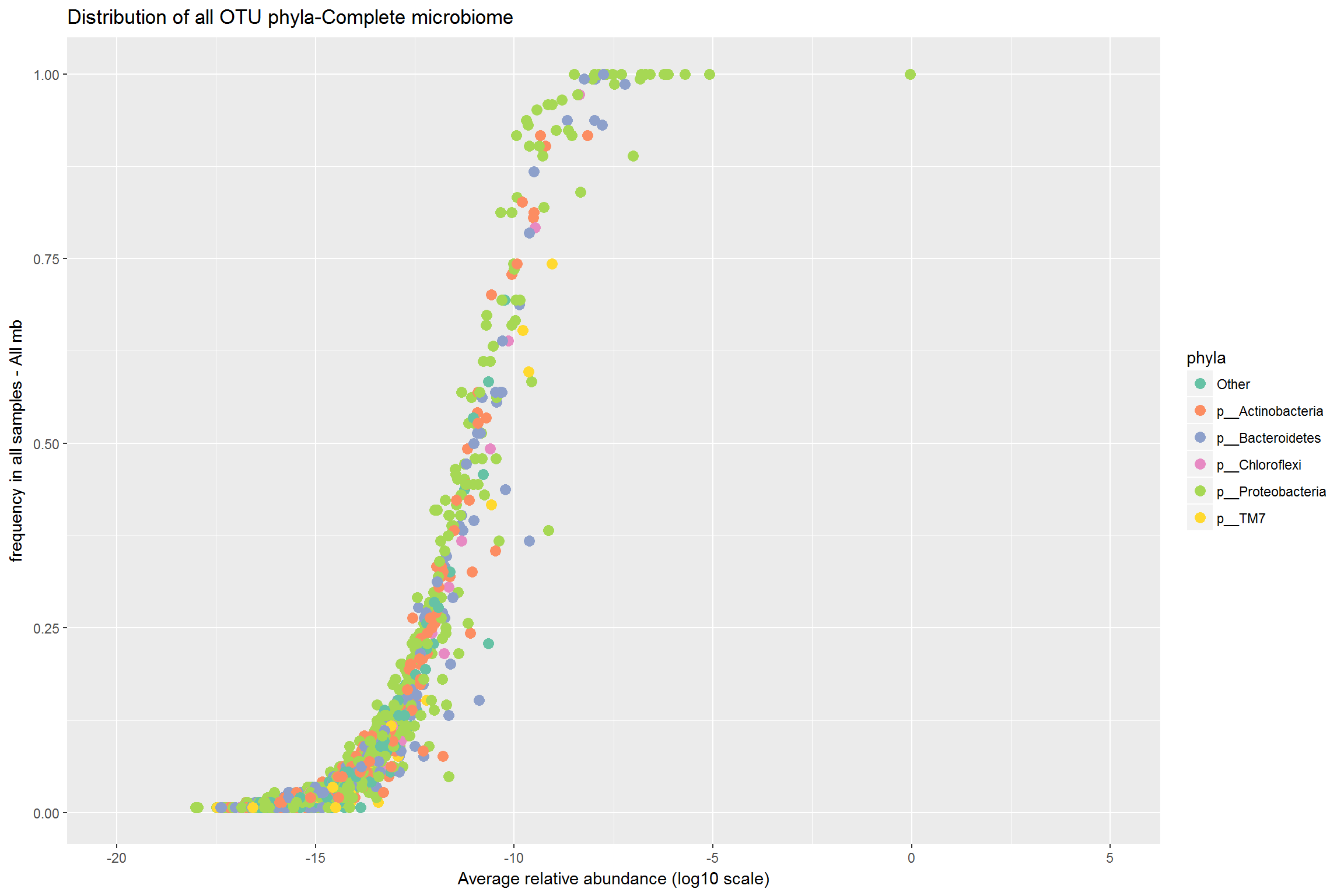``ggplot(abun_rare, aes(x=abundance,y=frequency,color=phyla)) + geom_point(size=3) + xlab("Average relative abundance (log10 scale)") + ylab("frequency in all samples - Rare mb") + scale_colour_brewer(palette="Set2")+labs(title="Distribution of all OTU phyla-Rare microbiome")+ xlim(-20, 5)``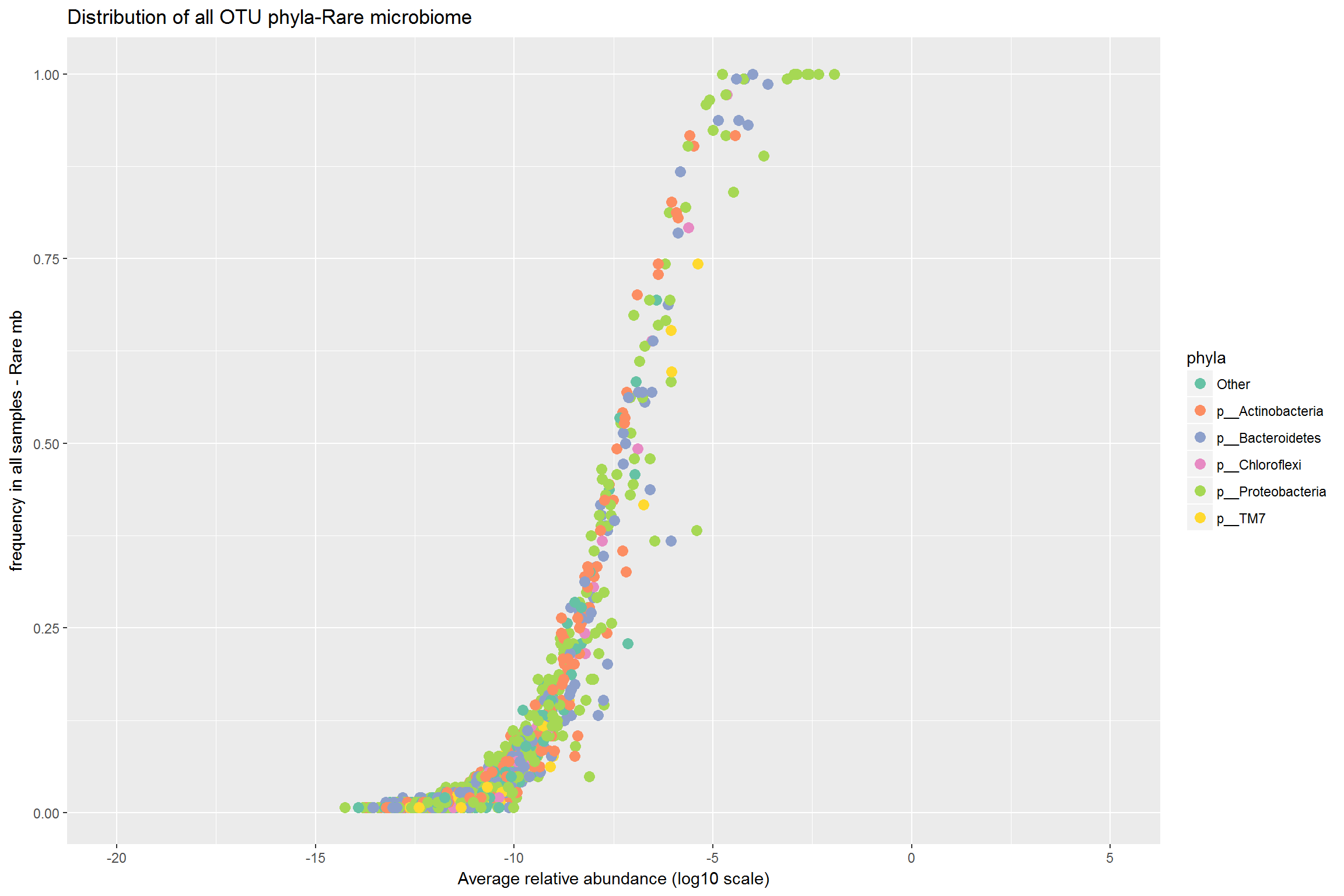``ggplot(abun_rhiz, aes(x=abundance,y=frequency,color=phyla)) + geom_point(size=3) + xlab("Average relative abundance (log10 scale)") + ylab("frequency in all samples - Rhiz mb") + scale_colour_brewer(palette="Set2")+labs(title="Distribution of all OTU phyla-Rhizobiales microbiome")+ xlim(-20, 5)``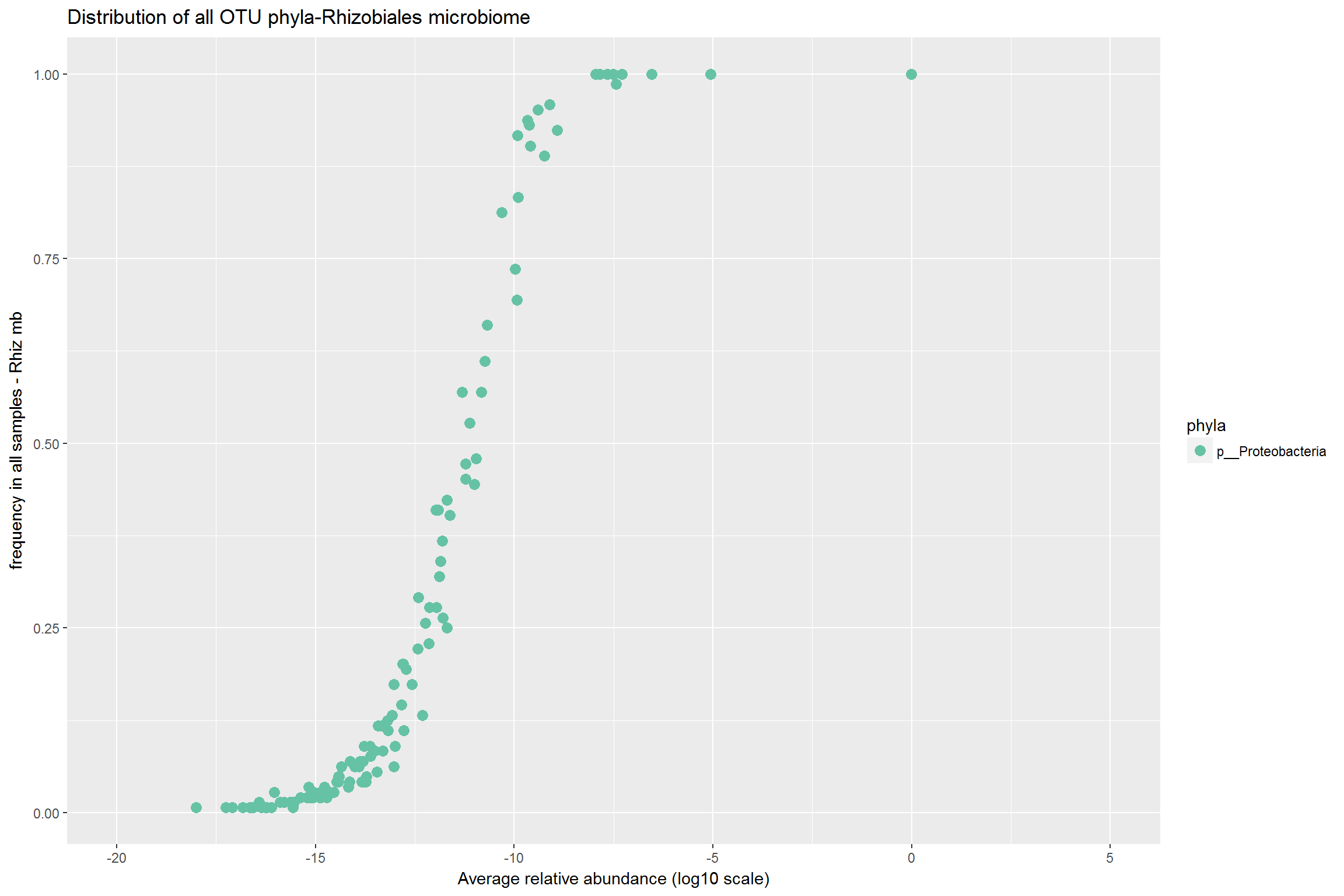``#bar plots needed for rare and rhizobiales taxa ``

### 7) Alpha diversity

We make use of three species diversity metrics to evaluate alpha diversity. Observed species, Shannon and Chao1. All OTU tables were rarefied to 160 and 6000 reads per samples. Soil and species do crop out as important factors from anova but the strength is largest for the smaller samples and also the results are not consistent. Under some metrics we see a significant effect while in others we dont. This is mostly due to an issue of undersampling. There is no correct way to proceed from here.

``````adiv_anova <- function(ss_no, otu_table){
mlist <- factor(c("Observed", "Shannon", "Chao1"))
set.seed(3336)
rarefied <- rarefy_even_depth(otu_table, sample.size = ss_no)
richness <- estimate_richness(rarefied, measures=mlist)
richness <- richness[-3]
sdata <- sample_data(rarefied)
for ( i in 1:3){
aov_facs <- aov(richness[[i]]~ sdata\$Soil*sdata\$Species)
print(summary(aov_facs))
}
}

adiv_anova(160, mb_rare)## all factors crop out as significant. This disappears at higher rarefactions``````
``````##                           Df Sum Sq Mean Sq F value  Pr(>F)
## sdata\$Soil                 5  495.8   99.16   3.534 0.00535 **
## sdata\$Species              5  592.5  118.49   4.224 0.00152 **
## sdata\$Soil:sdata\$Species  25 1660.6   66.42   2.368 0.00122 **
## Residuals                108 3030.0   28.06
## ---
## Signif. codes:  0 '***' 0.001 '**' 0.01 '*' 0.05 '.' 0.1 ' ' 1
##                           Df Sum Sq Mean Sq F value   Pr(>F)
## sdata\$Soil                 5  11388  2277.6   4.520 0.000884 ***
## sdata\$Species              5   5869  1173.8   2.329 0.047328 *
## sdata\$Soil:sdata\$Species  25  20937   837.5   1.662 0.039255 *
## Residuals                108  54423   503.9
## ---
## Signif. codes:  0 '***' 0.001 '**' 0.01 '*' 0.05 '.' 0.1 ' ' 1
##                           Df Sum Sq Mean Sq F value Pr(>F)
## sdata\$Soil                 5  0.393 0.07851   1.341 0.2525
## sdata\$Species              5  0.734 0.14688   2.509 0.0344 *
## sdata\$Soil:sdata\$Species  25  2.406 0.09624   1.644 0.0426 *
## Residuals                108  6.323 0.05854
## ---
## Signif. codes:  0 '***' 0.001 '**' 0.01 '*' 0.05 '.' 0.1 ' ' 1``````
``adiv_anova(160, mb_rhiz)``
``````##                           Df Sum Sq Mean Sq F value Pr(>F)
## sdata\$Soil                 5   9.62  1.9236   2.481 0.0362 *
## sdata\$Species              5   1.28  0.2569   0.331 0.8931
## sdata\$Soil:sdata\$Species  25  33.67  1.3469   1.737 0.0278 *
## Residuals                108  83.75  0.7755
## ---
## Signif. codes:  0 '***' 0.001 '**' 0.01 '*' 0.05 '.' 0.1 ' ' 1
##                           Df Sum Sq Mean Sq F value Pr(>F)
## sdata\$Soil                 5  31.40   6.281   2.297 0.0501 .
## sdata\$Species              5   4.59   0.918   0.336 0.8902
## sdata\$Soil:sdata\$Species  25 106.15   4.246   1.553 0.0638 .
## Residuals                108 295.31   2.734
## ---
## Signif. codes:  0 '***' 0.001 '**' 0.01 '*' 0.05 '.' 0.1 ' ' 1
##                           Df  Sum Sq  Mean Sq F value Pr(>F)
## sdata\$Soil                 5 0.01678 0.003355   1.679 0.1458
## sdata\$Species              5 0.00372 0.000744   0.372 0.8666
## sdata\$Soil:sdata\$Species  25 0.08266 0.003306   1.655 0.0406 *
## Residuals                108 0.21581 0.001998
## ---
## Signif. codes:  0 '***' 0.001 '**' 0.01 '*' 0.05 '.' 0.1 ' ' 1``````
``adiv_anova(6000, mb_rare)``
``````##               Df Sum Sq Mean Sq F value Pr(>F)
## sdata\$Soil     3  11041    3680   9.389  0.234
## sdata\$Species  1    313     313   0.797  0.536
## Residuals      1    392     392
##               Df Sum Sq Mean Sq F value Pr(>F)
## sdata\$Soil     3  13286    4429  29.834  0.134
## sdata\$Species  1    568     568   3.828  0.301
## Residuals      1    148     148
##               Df  Sum Sq Mean Sq F value Pr(>F)
## sdata\$Soil     3 0.13982 0.04661   0.251  0.860
## sdata\$Species  1 0.03055 0.03055   0.164  0.755
## Residuals      1 0.18591 0.18591``````
``adiv_anova(6000, mb_rhiz)``
``````##                           Df Sum Sq Mean Sq F value Pr(>F)
## sdata\$Soil                 5  124.1  24.828   2.882 0.0176 *
## sdata\$Species              5   34.5   6.894   0.800 0.5519
## sdata\$Soil:sdata\$Species  25  202.2   8.088   0.939 0.5532
## Residuals                108  930.5   8.616
## ---
## Signif. codes:  0 '***' 0.001 '**' 0.01 '*' 0.05 '.' 0.1 ' ' 1
##                           Df Sum Sq Mean Sq F value Pr(>F)
## sdata\$Soil                 5    732   146.5   1.211  0.309
## sdata\$Species              5    659   131.7   1.089  0.371
## sdata\$Soil:sdata\$Species  25   3760   150.4   1.243  0.220
## Residuals                108  13066   121.0
##                           Df   Sum Sq   Mean Sq F value Pr(>F)
## sdata\$Soil                 5 0.003124 0.0006247   2.191 0.0604 .
## sdata\$Species              5 0.004012 0.0008025   2.814 0.0198 *
## sdata\$Soil:sdata\$Species  25 0.007348 0.0002939   1.031 0.4357
## Residuals                108 0.030796 0.0002851
## ---
## Signif. codes:  0 '***' 0.001 '**' 0.01 '*' 0.05 '.' 0.1 ' ' 1``````

### 8) Beta diversity: Ordinations

The scree plot shows the number of dominant factors explaining variance. In both the complete and abundant table we see that scree plot has a single dominant axis. however when we look at the scree plot for only the rare microbiome, we see that there are multiple dominant axis with the first two explaining the greatest variance. This indicates that scree plot and ordinations for both complete and abundant tables are driven by the abundant, dominant OTU. Labelling and coloring of arrows for the CCA graphs can be improved under inkscape.

This section needs work

``````#Ordinations: PCoA principal
mb.pcoa.all <- ordinate(norm_all, d_all, method="PCoA")
pcoa.all<-plot_ordination(norm_all, mb.pcoa.all, color="Soil", shape="Species")+geom_point(size=5)+labs(title="PCoA plot for all OTUs")
pcoa.all``````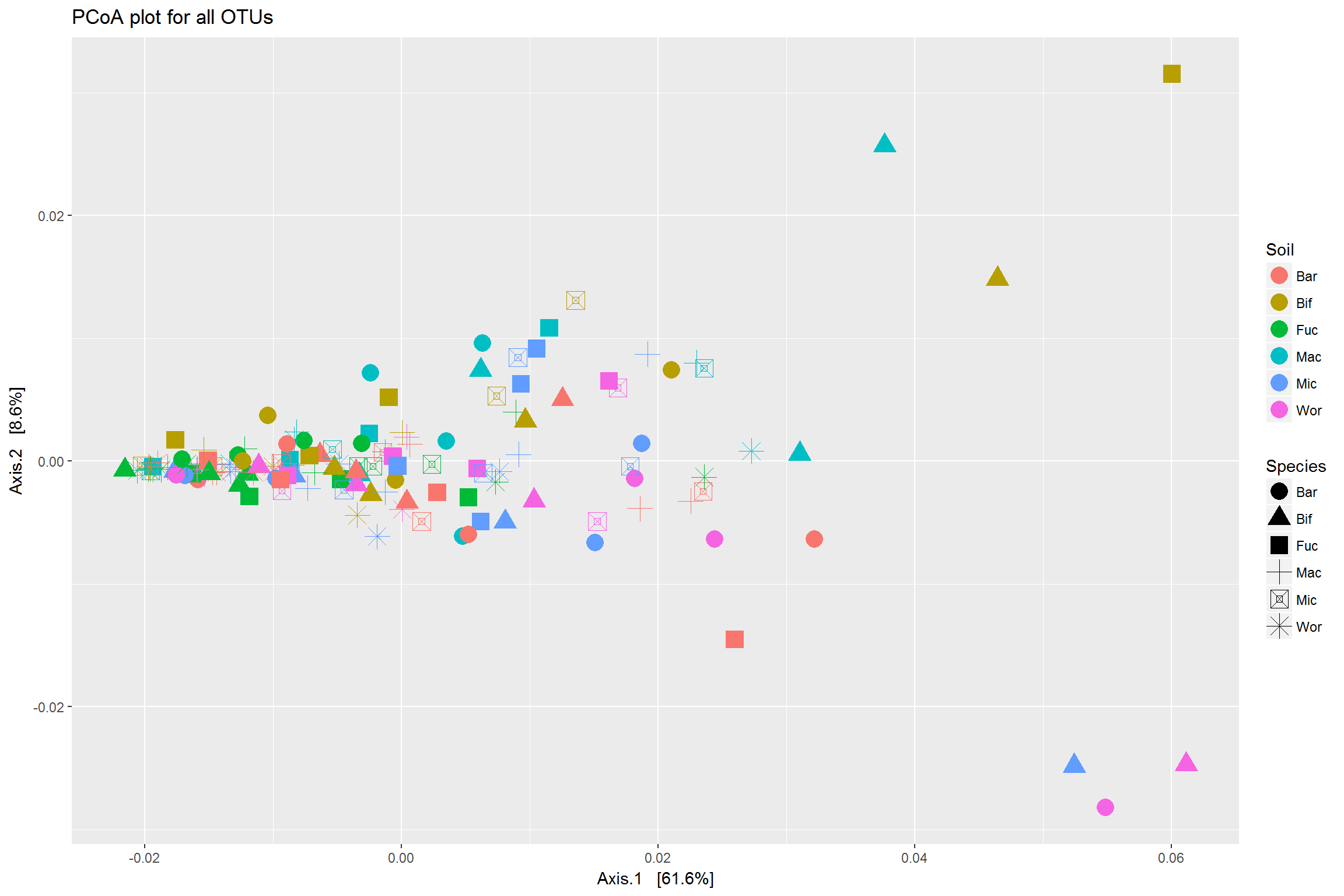``````scree <- head(mb.pcoa.all\$values["Eigenvalues"],20)
barplot(scree\$Eigenvalues, ylab="Eigenvalues")``````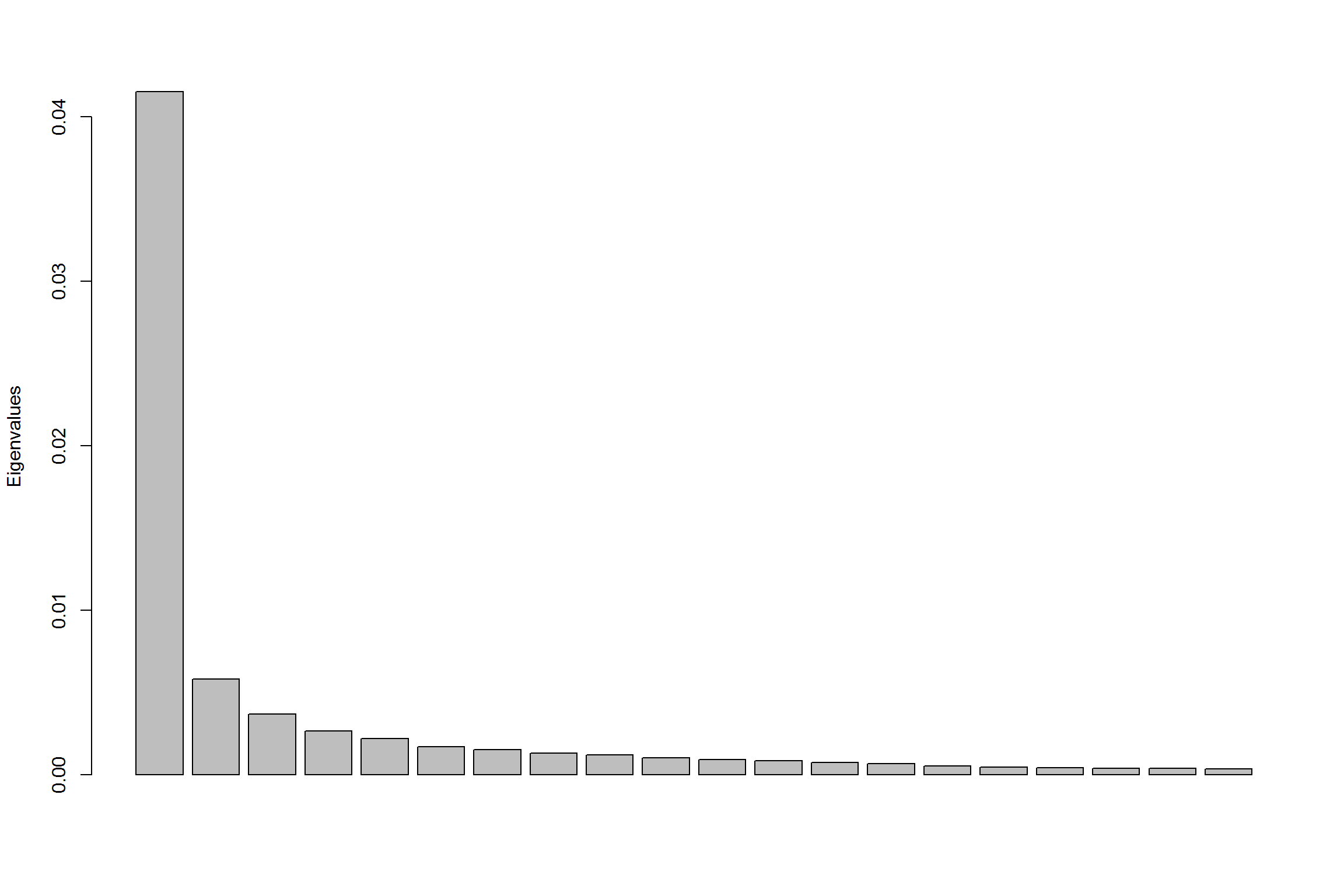``````mb.pcoa.rhiz <- ordinate(norm_rhiz, d_rhiz, method="PCoA")
pcoa.rhiz<-plot_ordination(norm_rhiz, mb.pcoa.rhiz, color="Soil", shape="Species")+geom_point(size=5)+labs(title="PCoA plot for Rhizobales OTUs")
pcoa.rhiz``````